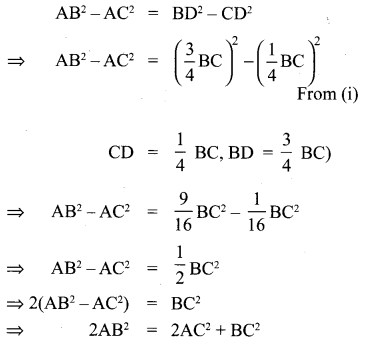# Samacheer Kalvi 10th Maths Solutions Chapter 4 Geometry Additional Questions

## Tamilnadu Samacheer Kalvi 10th Maths Solutions Chapter 4 Geometry Additional Questions

Question 1.
In figure if PQ || RS, Prove that ∆POQ ~ ∆SOR
Solution:
PQ || RS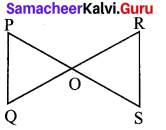So, ∠P = ∠S (A Hernate angles)
and ∠Q = ∠R
Also, ∠POQ = ∠SOR (vertically opposite angle)
∴ ∆POQ ~ ∆SOR (AAA similarity criterion)

Question 2.
In figure OA . OB = OC . OD Show that ∠A = ∠C and ∠B = ∠D
Solution:
OA . OB = OC . OD (Given)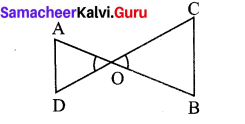Also we have ∠AOD = ∠COB
(vertically opposite angles) …………. (2)
From (1) and (2)
∴ ∆AOD ~ ∆COB (SAS similarity criterion)
So, ∠A = ∠C and ∠B = ∠D
(corresponding angles of similar triangles)

Question 3.
In figure the line segment XY is parallel to side AC of ∆ABC and it divides the triangle into two parts of equal areas. Find the ratio $$\frac{\mathbf{A X}}{\mathbf{A B}}$$
Solution:
Given XY||AC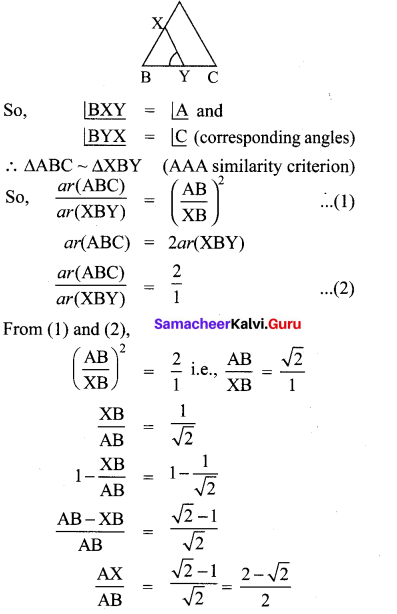Question 4.
In AD⊥BC, prove that AB2 + CD2 = BD2 + AC2.
Solution:
AC2 = AD2 + CD2 …………… (1)
(Pythagoras theorem)
AB2 = AD2 + BD2 …………. (2)
(Pythagoras theorem)
Subtracting (1) from (2) we have,
AB2 – AC2 = BD2 – CD2
AB2 + CD2 = BD2 + AC2Question 5.
BL and CM are medians of a triangle ABC right angled at A.
Prove that 4(BL2 + CM2) = 5BC2.
Solution:
BL and CM are medians at the ∆ABC in which
A = ∠90°.
From ∆ABC
BC2 = AB2 + AC2 …………… (1)
(Pythagoras theorem)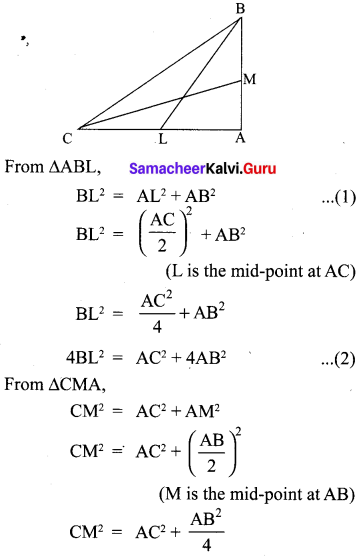4CM2 = 4AC2 + AB2 …………… (3)
Adding (2) and (3), we have
4(BL2 + CM2) = 5(AC2 + AB2)
4(BL2 + CM2) = 5BC2 [From (1)]

Question 6.
Prove that in a right triangle, the square of the hypotenure is equal to the sum of the squares of the others two sides.
Solution:
Proof: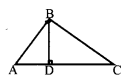We are given a right triangle ABC right angled at B.
We need to prove that AC2 = AB2 + BC2
Let us draw BD ⊥ AC
$$\frac{\mathrm{AD}}{\mathrm{AB}}=\frac{\mathrm{AB}}{\mathrm{AC}}$$ (sides are proportional)
AD . AC = AB2 …………. (1)
Also, ∆BDC ~ ∆ABC
$$\frac{C D}{B C}=\frac{B C}{A C}$$
CD . AC = BC2 …………. (2)
AD . AC + CD . AC = AB2 + BC2
AC(AD + CD) = AB2 + BC2
AC . AC = AB2 + BC2
AC2 = AB2 + BC2Question 7.
A ladder is placed against a wall such that its foot is at a distance of 2.5 m from the wall and its top reaches a window 6 m above the ground. Find the length of the ladder.
Solution:
Let AB be the ladder and CA be the wall with the window at A.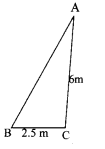Also, BC = 2.5 m and CA = 6 m
From Pythagoras theorem,
AB2 = BC2 + CA2
= (2.5)2 + (6)2
= 42.25
AB = 6.5
Thus, length at the ladder is 6.5 m.

Question 8.
In figure O is any point inside a rectangle ABCD. Prove that OB2 + OD2 = OA2 + OC2.
Solution:
Through O, draw PQ||BC so that P lies on AB and Q lies on DC.
Now, PQ||BC
PQ⊥AB and PQ⊥DC (∵ ∠B = 90° and ∠C = 90°)
So, ∠BPQ = 90° and ∠CQP = 90°
Therefore BPQC and APQD are both rectangles.
Now from ∆OPB,
OB2 = BP2 + OP2 ……………. (1)
Similarly from ∆OQD,
OD2 = OQ2 + DQ2 …………. (2)
From ∆OQC, we have
OC2 = OQ2 + CQ2 ……….. (3)
∆OAP, we have
OA2 = AP2 + OP2 …………. (4)
OB2 + OD2 = BP2 + OP2 + OQ2 + DQ2 (As BP = CQ and DQ = AP)
= CQ2 + OP2 + OQ2 + AP2
= CQ2 + OQ2 + OP2 + AP2
= OC2 + OA2 [From (3) and (4)]Question 9.
In ∠ACD = 90° and CD⊥AB. Prove that $$\frac{\mathbf{B C}^{2}}{\mathbf{A C}^{2}}=\frac{\mathbf{B D}}{\mathbf{A D}}$$
Solution:
∆ACD ~ ∆ABC
So,Question 10.
The perpendicular from A on side BC at a ∆ABC intersects BC at D such that DB = 3 CD. Prove that 2AB2 = 2AC2 + BC2.
Solution:
We have DB = 3 CD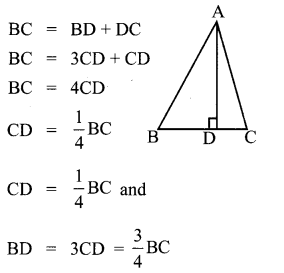Since ∆ABD is a right triangle (i) right angled at D
AB2 – AD2 + BD2 …………. (ii)
By ∆ACD is a right triangle right angled at D
AC2 = AD2 + CD2 ………… (iii)
Subtracting equation (iii) from equation (ii),
we got